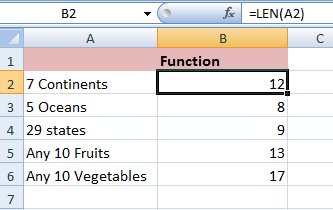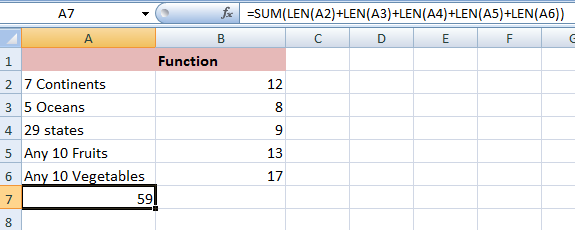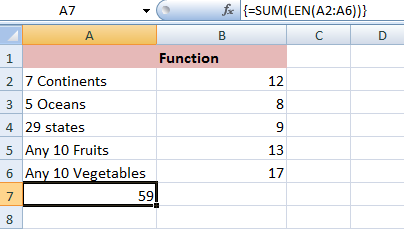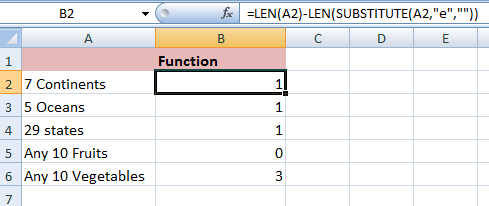### Count Characters in MS Excel

How to Count Characters in MS Excel
To Count the Characters in the Cell, LEN function is used.
**  LEN Function counts letters, numbers, characters, and all spaces.
Let's learn with Example
For example, for counting the Characters in cell A2 , the Function will be B2 equals =LEN(A2)
Here, LEN function counts 1 number, 1 space and 10 letters.Likewise, It can be calculated for all the cells.
>> The SUM function and the LEN function can be combined to count the number of characters in selected range of cells.
For example, for counting the Characters in Range A2:A6 ,
Function will be A7 equals  =SUM(LEN(A2)+LEN(A3)+LEN(A4)+LEN(A5)+LEN(A6))## As this Formula can be as long as the selected Range. The array formula counts the number of characters in range of cells.
For example, for counting the Characters in Range A2:A6 ,
Function will be A7 equals =SUM(LEN(A2:A6))
** Always finish Array Formula by pressing CTRL+SHIFT+ENTER. Excel will automatically add the curly braces {}.>> The SUBSTITUTE function and the LEN function can be combined to count, how many times a specific character occurred in the specific cell.
For example, for counting the occurrence of characters (e) in cell A2
Function will be B2 equals =LEN(A2)-LEN(SUBSTITUTE(A2,"e",""))
** SUBSTITUTE function replaces the character (e) (second argument) with an empty string (third argument). LEN(SUBSTITUTE(A2,"e","")) equals 11 (the length of the string without the character e). If we subtract this number from 12 (total number of characters in cell A2), we get the number of occurrences of the character e in cell A2.Likewise, It can be calculated for all the cells.
## As this Formula can be as long as the selected Range. The array formula counts the specific number of characters in selected range of cells.
For example, for counting the Character (e) in Range A2:A6,
Function will be A7 equals =SUM(LEN(A2:A6)-LEN(SUBSTITUTE(A2:A6,"e","")))
** Always finish Array Formula by pressing CTRL+SHIFT+ENTER. Excel will automatically add the curly braces {}. The SUBSTITUTE function is case-sensitive. So, if any Text has E, it will not be Counted.